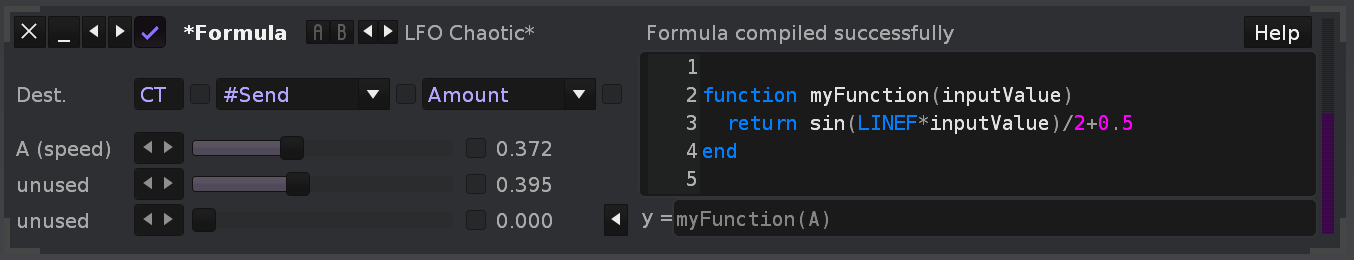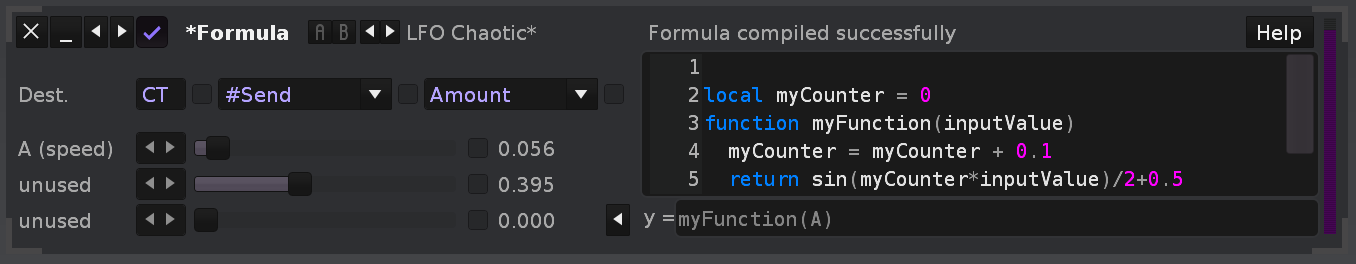# Formula Device: How To Learn To Write Formulas

I want to experiment with the Formula Device but I don’t know how to begin.

I understand the premise of the device. It’s take inputs, runs formulas, and generates an output based on those formulas that can be mapped to a desired location. No problems there.

My issue is with the actual creation of the formulas themselves. I have no idea how to write them or how to get started writing them. I’ve read the “help” menu details in the device with all the listed “variables” “math constants” “musical variables” & “functions” and I can grasp their purpose in that they are what is used to create the formulas, but again, I have no idea how to actually write my own formulas using them.

What do I need to study so I can get started with this?

Do I need to learn a specific type of math used for DSP math like trigonometry? Do I need to learn the LUA programming language to write formulas? And if so are there any specific resources like websites, books, etc, that I should refer to learn from?

Thanks

8 Likes

thank you for writing a question that i had really hard time writingfollowing this

3 Likes

I too would like to understand this - I am curious to see what applications of the module the devs had in mind when implementing it. Can it be used to multiply one signal by another and take us one step closer a potential native FM?

Watching also.

2 Likes

Also interested!

Pretty sure the formula device can’t manipulate audio directly, so I don’t think it can be used for native fm/pm - although that would be great, of course, if it could.

3 Likes

A basic (audio) signal processing tutorial might help, also read about floating point numbers, and basic functions. Here it is about meta signals, which range unipolar from 0 to 1 value-range, instead like audio signals in the range -1 to 1. Still both are similar. You often can use an “audio dsp” approach and convert the output signal’s range then simply to the unipolar one (e.g. division by 2 and then addition by 0.5).

Since the value range is only from 0 to 1, the math usually is pretty basic. Set yourself some simple exercises, for example: “I want to create a sine meta signal with the frequency as single parameter”. Then usually it is a quite simple one liner equation (very basic implementation):The three input sliders (which you can name as you want) always have the variable names A, B and C. In the y= field you can also call any custom function which you wrote into the code editor above.

LINEF is the pattern position while playback. You also could implement an own counter instead, so your basic LFO was not dependent on song position anymore:The equation of the formula device seems to be re-calculated on a statical time interval all the time, no matter what. Hope that helps somehow…

6 Likes

Yeah this helps a bit, I’m grasping the concept of it. But I’m gonna need more resources than this to learn from.

So the formulas aren’t written in LUA code then? (Bear in my mind I don’t know LUA or what it even looks like, I’ve only heard about it so that’s why I’m confused right now) They are written out using mathematical functions that can be learned through studying Audio Signal Processing yes? Is that the same thing as Digital Signal Processing?

Is there anywhere I can go to find more examples like this to study and learn from, websites, books, etc?

Yes the formula device uses LUA. So learning some basic (procedural, not necessarily object oriented) LUA knowledge is necessary for using the device. I’d go for basic syntax like hello world, variables and formulas, functions, arrays, the math library, conditions and loops, and then you already know enough to use the device to full effect.

It executes at each tick the “y =” formula line, which is to be a LUA formula to calculate the output value. It implies the “y=” part, so the formula must return a number value. You can use functions and variables in that line that you declare in the code field. Whatever is written in the code field will get executed once at startup or when the content is changed, then the formula field seems to get called for each tick and for each input value change. Try a formula “A+B” and move the first two sliders to see what happens, to get an idea…

The output must be in the range between 0.0 and 1.0, and can be used to control an effect/plugin automation parameter. So really it is really mostly only useful for automation, but you can program the automation in very custom ways with it. The A,B,C parameters are three effect parameters (also 0.0…1.0) of the formula that can be used to automate the formula itself (but you don’t have to use them), and they are available as variables in the code you write. The “help” field just lists the available variables and functions. Thus you can do calculations based on current line number, BPM and stuff like that.

Note that it is stripped down LUA, it does not have all the functions and stuff available that normal LUA has (for example when writing renoise tools). But I think the formula device is a nice little toy to get started with LUA programming. It is not easy to debug though. The code will be executed immediately when you change something, and will be effective right away…if there is a syntax error, the device will stop working and show an error message above the code field. But if your code is wrong somehow but has no syntax errors, it will not detect that, and the formula might not work as expected…

So…to get started, you first need a proper use case you wish to program. Like some special kind of automation that you would like to have going on automatically. Like for example a custom LFO that changes behavior dependent on the value from a velocity tracker, or from the line number that is currently playing… Just think - it will control one parameter, and you can use three inputs to define what is done. The rest ist subject to math/programming. It can be very simple, or very complicated…in the end it is just LUA programming to achive what is needed.

And no, it cannot be used for FM/PM, it is bound to controlling plugin parameters at tick rate. So it is rather an automation tool. Chaining multiple formula devices can result in interesting possibilities…or playful things, like I once put work into that a formula device can have more than three inputs parameters by mathematically compressing numbers into a single value, or to translate pattern FX commands controlling the A,B,C sliders into actions that the formula device will execute, like retriggering an LFO at certain positions, or setting the speed to exact values. That is what I mostly use it for. Other people seem to rather use it for procedural music kind of things, where programmed automation yields mathematically controlled audio landscapes. Just saying, for your inspiration on what to try…

12 Likes

Fantastic. Thanks for such a detailed answer. Now that I know it’s LUA for definite I have a clear starting point from where to work with which is brilliant.

Really appreciate it, thanks again.

2 Likes
1 Like

Nice!! The official LUA website also has a book hosted on it for free Programming in Lua (first edition)

1 Like

Sweet. I’ll have to go thru a tutorial or two and see if it’s clear as mud, or whatSometimes a hard copy for reference makes a big difference!

don’t forget when you use math functions like sin cos you can omit the trailing “math.” as the formula device will automatically load that namespace. This is different in other lua environments where you might like to test some buggy code. There you have to do:

``````sin = math.sin
cos = math.cos
sqrt = math.sqrt
...``````

to be able to use the same code

3 Likes

Throw in instructions for this, maybe a link. or am I misunderstanding something

I was referencing this ^ and this:
https://www.tutorialspoint.com/lua/index.htm

welcome to the forum!